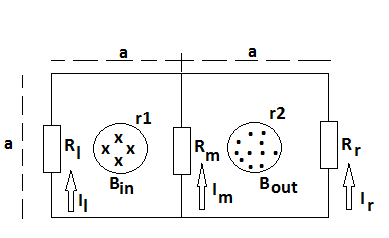# Two infinitely long solenoids thread a circuit. The magnitude of B inside each solenoid is the...

## Question:

Two infinitely long solenoids thread a circuit. The magnitude of {eq}B {/eq} inside each solenoid is the same and is {eq}300\ T {/eq} at time {eq}t = 0 {/eq}.

What is the magnitude of current {eq}I_m {/eq} in middle resistor {eq}R_m {/eq} ? {eq}a = 0.7\ m, r_1 = 0.2\ m, r_2 = 0.1\ m, \frac{\Delta B}{\Delta t} = 140\ T/s, R_l = 8.6\ \Omega, R_m = 4.3\ \Omega \ and \ R_r = 2.7 \ \Omega. {/eq}If a magnetic field flux is changing in a region in space, the changing magnetic field flux induces electric field around it, which is proportional to the rate of change in magnetic field flux. The direction of induced emf is such that it opposes the change in magnetic field flux that induced it.

According to the Faraday's Law, the changing magnetic field flux induces a circular electric field, or electromotive force, around itself. We will assume increasing magnetic field, for the decreasing magnetic field the results are the same but all current directions will be reversed. Increasing magnetic field will induce emf directed counterclockwise for the left contour and clockwise for the right one. The magnitude of the induced emf is given by Faraday's Law:

{eq}\mathscr{E} = \dfrac {\Delta \Phi}{\Delta t} = \dfrac {\Delta B}{\Delta t}\pi r^2 {/eq}

For the given circuit, we can write the following system of equations using Kirchhoff's rules (mind the direction of emf, stated above!):

{eq}\begin{align*} & \pi r^2_1 \dfrac {\Delta B}{\Delta t} = I_m R_m - I_lR_l &\text (1) \\ & \pi r^2_2 \dfrac {\Delta B}{\Delta t} = I_m R_m - I_rR_r &\text (2) \\ &I_m + I_r + I_l = 0 &\text (3) \end{align*} {/eq}

Eliminating the current through the right resistor, we obtain:

{eq}\begin{align*} & \pi r^2_1 \dfrac {\Delta B}{\Delta t} = I_m R_m - I_lR_l &\text (4) \\ & \pi r^2_2 \dfrac {\Delta B}{\Delta t} = I_m R_m + (I_m + I_l)R_r = I_m(R_m + R_r) + I_l R_r &\text (5) \\ \end{align*} {/eq}

Multiplying the first equation by {eq}R_r {/eq}, the second one by {eq}R_l {/eq}, and adding them, we obtain:

{eq}\dfrac {\Delta B}{\Delta t} \pi (r^2_2 R_l + r^2_1 R_r) = I_m (R_m R_l + R_rR_l + R_m R_r) {/eq}

Solving for the current, we get:

{eq}I_m = \dfrac {\dfrac {\Delta B}{\Delta t} \pi (r^2_2 R_l + r^2_1 R_r)}{(R_m R_l + R_rR_l + R_m R_r)} {/eq}

Calculation yields:

{eq}I_m = \dfrac {140 \ T/s \times \pi \times ((0.1 \ m)^2 \times 8.6 \Omega + (0.2 \ m)^2 \times 2.7 \ \Omega)}{(4.3 \ \Omega \times 8.6 \Omega + 2.7 \ \Omega \times 8.6 \Omega + 4.3 \ \Omega \times 2.7 \ \Omega)} \approx \boxed {0.38 \ A} {/eq}